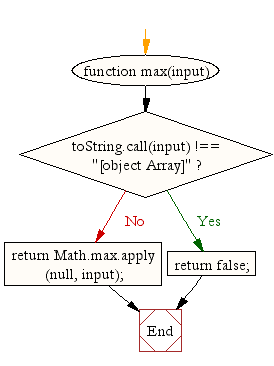# JavaScript: Find the highest value in an array

## JavaScript Math: Exercise-6 with Solution

Write a JavaScript function to find the highest value in an array.

Test Data :
console.log(max([12,34,56,1]));
console.log(max([-12,-34,0,-56,-1]));
56
0

Pictorial Presentation:Sample Solution:-

HTML Code:

``````<!DOCTYPE html>
<html>
<meta charset="utf-8">
<title>Find the highest value in an array</title>
<body>

</body>
</html>
```
```

JavaScript Code:

``````function max(input) {
if (toString.call(input) !== "[object Array]")
return false;
return Math.max.apply(null, input);
}

console.log(max([12,34,56,1]));
console.log(max([-12,-34,0,-56,-1]));
```
```

Sample Output:

```56
0
```

Flowchart:Live Demo:

See the Pen javascript-math-exercise-6 by w3resource (@w3resource) on CodePen.

Improve this sample solution and post your code through Disqus

What is the difficulty level of this exercise?

Test your Programming skills with w3resource's quiz.

﻿